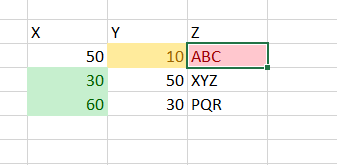# Multiply one column value to 2 values of other column

Hello Knime experts!

I am fairly new to KNIME and have started some work on this platform very recently.
I really need help with the below to progress further.
I am stuck at a stage, where I want to multiple one value of a column to the few values of second column.
Taking an examples in below figure:In this image I want to multiply one particular value from Y column which matches a condition on Z column (here is Z value is “ABC”, we choose that particular value of Y which is 10) with two values of X column say second and third values (these values are also chosen as per Z column value. So if Z value is XYZ , X taken is 30 and if Z value is PQR then X value taken is 60) separately.
So what I want is 1030=300 and 1060= 600 in two new columns.
here, selecting 10 value from Y column is as per match criteria of Z
and selecting 30 and 60 values is also as per match criteria of Z

• It is not required that Y column value to be used is first entry only, it can be any entry which I choose on a match condition of another column. eq, take value of Y where say col Z is “ABC”
• It is not required that X column value will be two values simultaneously, it can be any two from a set of rows as per match condition on Z column.
• Also, I can retrieve any number of values from X column to multiply with that specific value of Y column (I can have 3-4 values in X column which needs to be multiplied by 10 and result put under 3-4 new columns respectively).

I basically want to multiply one particular value from Y column (selected from a match condition on Z column) with some particular values of X column (also selected from match condition on Z column) and put that in new columns.

Hope to get some help with this.
Any help is much appreciated!

Hello @mahima_goyal,

following idea from given for your similar question in this topic additionally split your data (you can use Nominal Value Row Splitter node) based on Z condition and multiply with extracted value.

Br,
Ivan

Hello @ipazin
Thanks a lot for input but the solution in the topic worked for me.

Thanks alot!

This topic was automatically closed 7 days after the last reply. New replies are no longer allowed.